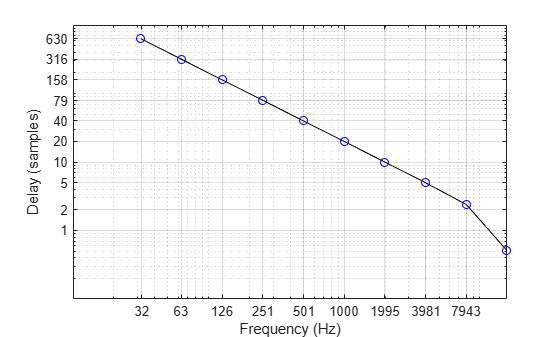# getGroupDelays

Get group delays

## Syntax

``groupDelays = getGroupDelays(obj)``
``[groupDelays,centerFrequencies] = getGroupDelays(obj)``

## Description

example

````groupDelays = getGroupDelays(obj)` returns the group delay of each filter at its center frequency.```
````[groupDelays,centerFrequencies] = getGroupDelays(obj)` returns the center frequency of each filter.```

## Examples

collapse all

Create a default `octaveFilterBank` object. Call `getGroupDelays` to get the group delay of each octave filter at its center frequency.

```octFiltBank = octaveFilterBank; [gd,cf] = getGroupDelays(octFiltBank);```

Plot the group delay as a function of filter center frequency.

```loglog(cf,gd,'k-',cf,gd,'bo') grid on xlabel('Frequency (Hz)') ylabel('Delay (samples)') xticks(round(cf)) yticks(round(fliplr(gd)))```## Input Arguments

collapse all

Object to get group delays from, specified as an object of `octaveFilterBank`.

## Output Arguments

collapse all

Group delay of each filter at its center frequency in samples, returned as a row vector.

Center frequencies of filters designed by `obj` in Hz, returned as a row vector.

Data Types: `double` | `single`